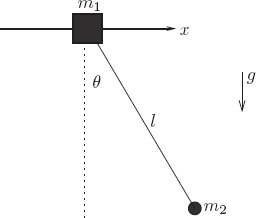### Exercise 1.20: Sliding pendulum

Consider a pendulum of length $l$ attached to a support that is free to move horizontally, as shown in the figure. Let the mass of the support be $m_1$ and the mass of the pendulum bob be $m_2$. Formulate a Lagrangian and derive Lagrange’s equations for this system.Figure 1.4

Consider the two bodies with their rectangular coordinates:

\begin{align} x_1 &= x \\ y_1 &= y \\ x_2 &= x + l\cos{\theta} \\ y_2 &= y + l\sin{\theta} \\ \end{align}

Use coordinate transform along with a constant-acceleration lagrangian for two particles to compute the equations for the system

(defn sliding-pend->rect [l]
(fn [[_ [x y theta] _]]
(up x
y
(+ x (* l (cos theta)))
(+ y (* l (sin theta)))
)))

(defn L-const-accel-two-bodies [m_1 m_2 g]
(fn [[_  [x_1 y_1 x_2 y_2] [v_x1 v_y1 v_x2 v_y2]]]
(let [T1 (* 1/2 (* m_1 (+ (square v_x1) (square v_y1))))
T2 (* 1/2 (* m_2 (+ (square v_x2) (square v_y2))))
T (+ T1 T2)
V1 (* m_1 g y_1)
V2 (* m_2 g y_2)
V (+ V1 V2)]
(- T V))))

(defn L-sliding-pend [m_1 m_2 g l]
(fn [q-prime]
((compose (L-const-accel-two-bodies m_1 m_2 g) (F->C (sliding-pend->rect l)))
q-prime)))

(def eom-sliding-pend
(let [L (L-sliding-pend 'm_1 'm_2 'g 'l)]
(Lagrange-equations L)
))

(rendertexvec
(let [state (up (literal-function 'x) (literal-function 'y) (literal-function 'theta))]
((eom-sliding-pend state) 't)))

\begin{pmatrix}\displaystyle{- l\,m_2\,\cos\left(\theta\left(t\right)\right)\,{\left(D\theta\left(t\right)\right)}^{2} - l\,m_2\,\sin\left(\theta\left(t\right)\right)\,{D}^{2}\theta\left(t\right) + m_1\,{D}^{2}x\left(t\right) + m_2\,{D}^{2}x\left(t\right)} \cr \cr \displaystyle{- l\,m_2\,{\left(D\theta\left(t\right)\right)}^{2}\,\sin\left(\theta\left(t\right)\right) + l\,m_2\,\cos\left(\theta\left(t\right)\right)\,{D}^{2}\theta\left(t\right) + g\,m_1 + g\,m_2 + m_1\,{D}^{2}y\left(t\right) + m_2\,{D}^{2}y\left(t\right)} \cr \cr \displaystyle{g\,l\,m_2\,\cos\left(\theta\left(t\right)\right) + {l}^{2}\,m_2\,{D}^{2}\theta\left(t\right) + l\,m_2\,\cos\left(\theta\left(t\right)\right)\,{D}^{2}y\left(t\right) - l\,m_2\,\sin\left(\theta\left(t\right)\right)\,{D}^{2}x\left(t\right)}\end{pmatrix}

← Back to workbook